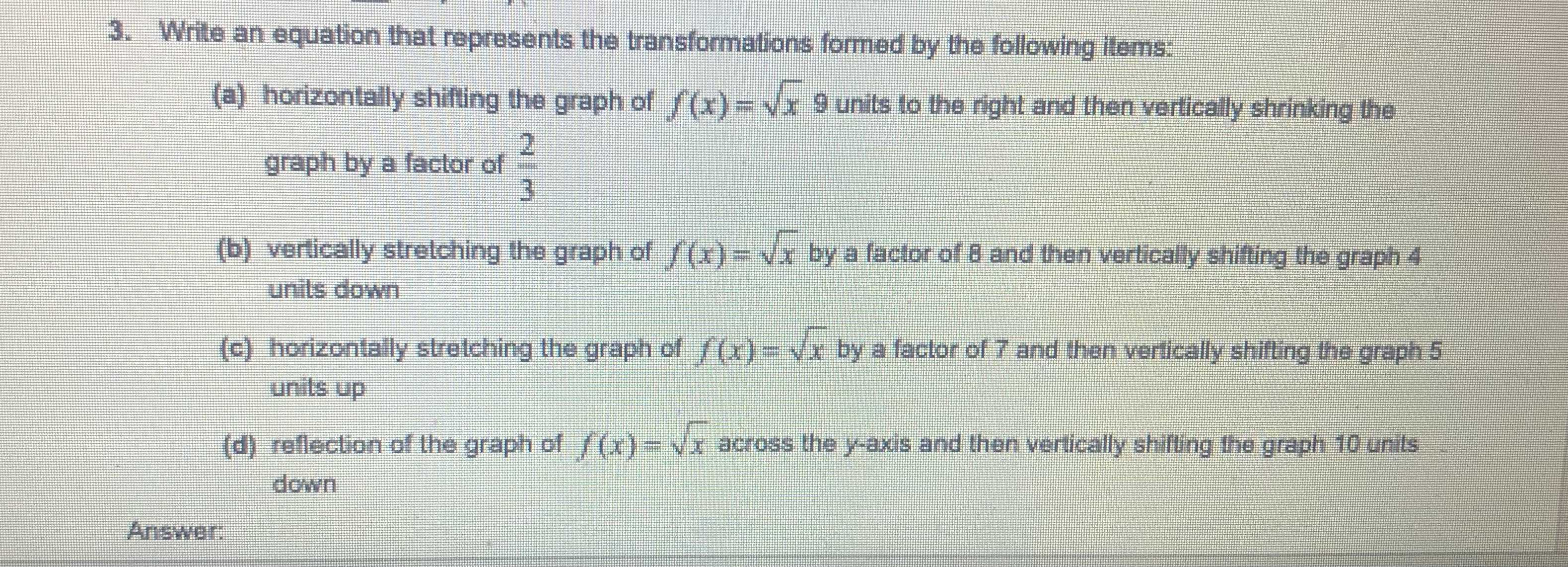### ¿Todavía tienes preguntas de matemáticas?

Pregunte a nuestros tutores expertos
Algebra
Pregunta3. Wrile an equation that represents the transformations formed by the following ilems:

(a) horizonlally shifing the graph of $$f ( x ) = \sqrt { x } 9$$ units lo the right and then vertically shrinking the graph by a factor of $$\frac { 2 } { 3 }$$

(b) vertically stretching the graph of $$f ( x ) = \sqrt { x }$$ by a faclor of $$8$$ and then vertically shiting the graph $$A$$ unis down

(c) horizonially stretching the graph of $$f ( x ) = \sqrt { x }$$ by a factor of $$7$$ and then verically shiting the graph $$5$$ units up

(d) rellection of the graph of $$f ( x ) = \sqrt { x }$$ across the y-axis and then verically shifting the graph $$10$$ unis down

$$(a)\sqrt{\frac{3}{2}(x- 9) }$$
$$(b)\sqrt{\frac{x}{8} } - 4$$
$$(c)\sqrt{\frac{x}{7} } + 5$$
$$(d)\sqrt{- x} - 10$$